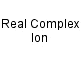Chromium(III) hexahydrated ion
[Cr(H2O)6]3+
Hexaaquachromium(III) ionCr 1 H 12 O 6 Charge = +3

Real ion.

Gram formula weight (molecular mass) = 160.08

The hexaaquachromium(III) ion is octahedral and is coloured red-violet.Hexaaquachromium(III) ion
is defined with respect to the entities below:
The entities below are defined with respect to:
Hexaaquachromium(III) ion
 Aqueous M(III) ion (AS/A2 generic)Complex (AS/A2 generic)Real complex ion (generic)Type 23 Lewis acid/base complex (generic)VSEPR geometry = AX6 (generic)Weak acid (AS/A2 generic)chemical compound molecule metal molecular science reaction mechanism ionic material acid base geometry reactivity synthesis science knowledge chemistry Lewis